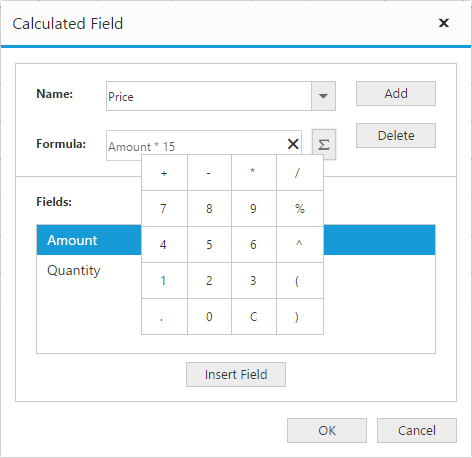# Calculated field

24 May 20191 minute to read

NOTE

This feature is applicable only for the relational data source.

The pivot grid provides support to insert a new calculated field based on the existing pivot fields through the Calculated Field dialog or code behind.

### Through UI

To insert a new calculated field, open the Calculated Field dialog by using the grouping bar context menu. You can define “Name” for the new calculated field, and “Formula” can be entered by inserting the required fields through the fields section. For inserting numbers and operators, you can use formula pop-up as shown in the following screenshot:Click Add for adding the respective calculated field, and click OK to populate the pivot grid control.

### Through code-behind

For client mode, the calculated field can be created at code-behind by defining the formula based on the existing pivot fields in the pivot grid. To indicate a field as a calculated field, set the [`is-calculated-field`] property to true and [`formula`] property to set the expression.

• CSHTML
• ``````<ej-pivot-grid id="PivotGrid1" enable-grouping-bar="true" load="onload">
<e-data-source>
<e-pivot-rows>
<e-row-field field-name="Country" field-caption="Country"></e-row-field>
</e-pivot-rows>
<e-pivot-columns>
<e-column-field field-name="Product" field-caption="Product"></e-column-field>
</e-pivot-columns>
<e-pivot-values>
<e-value-field field-name="Amount" field-caption="Amount" format="currency"></e-value-field>
<e-value-field field-name="Price" field-caption="Price" is-calculated-field="true" formula="Amount * 15"></e-value-field>
</e-pivot-values>
</e-data-source>
</ej-pivot-grid>``````